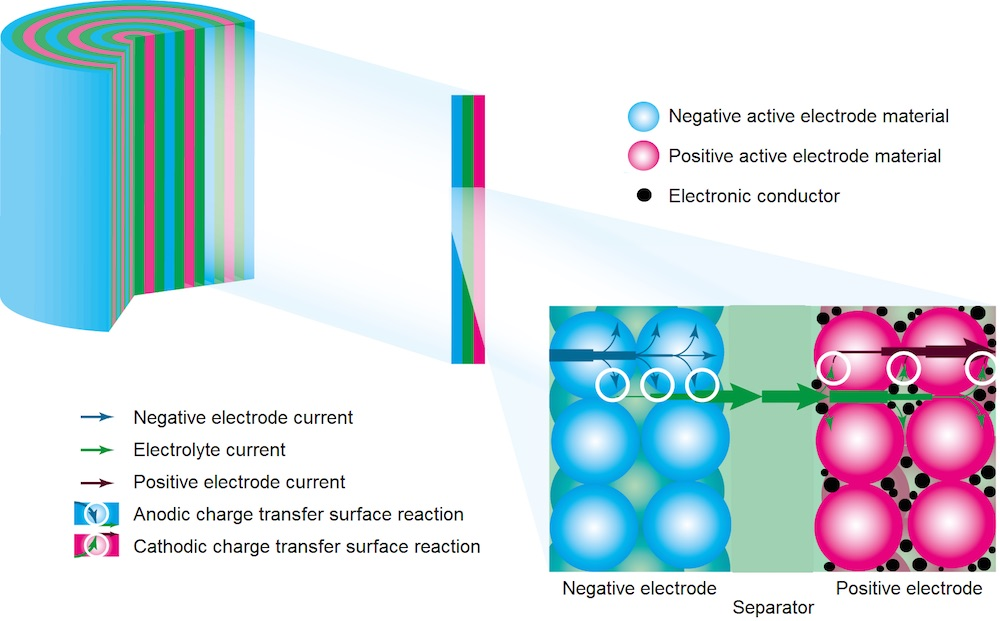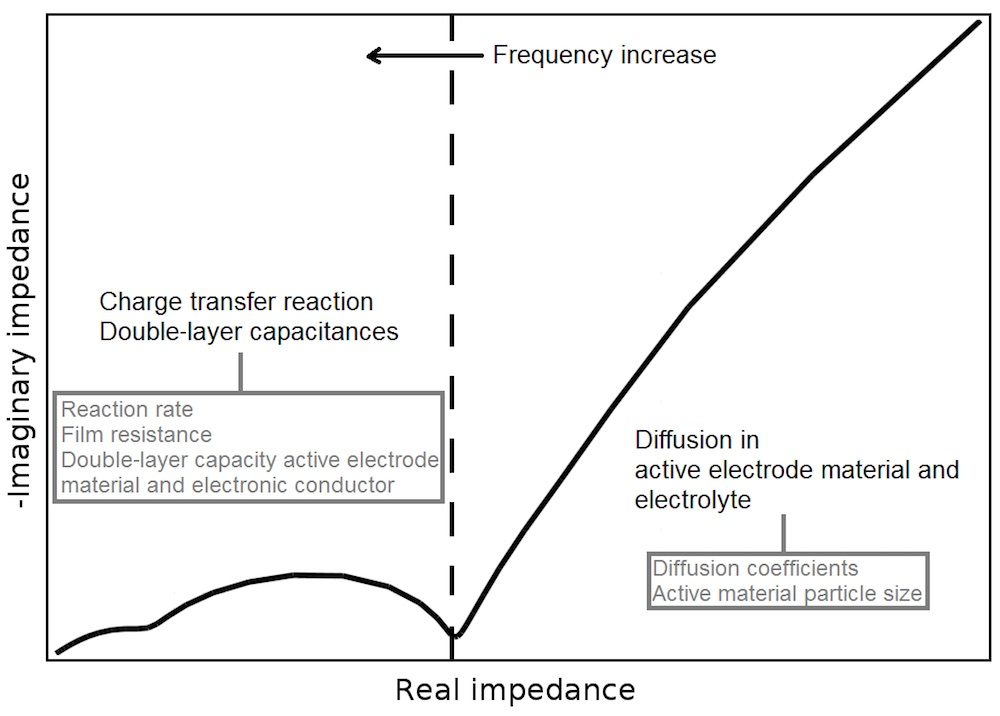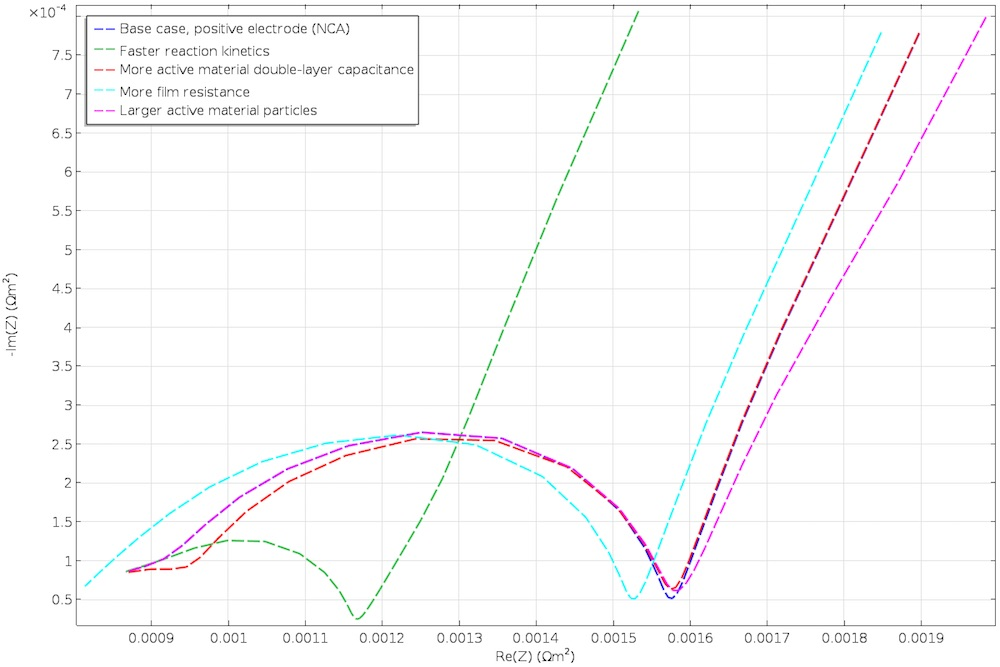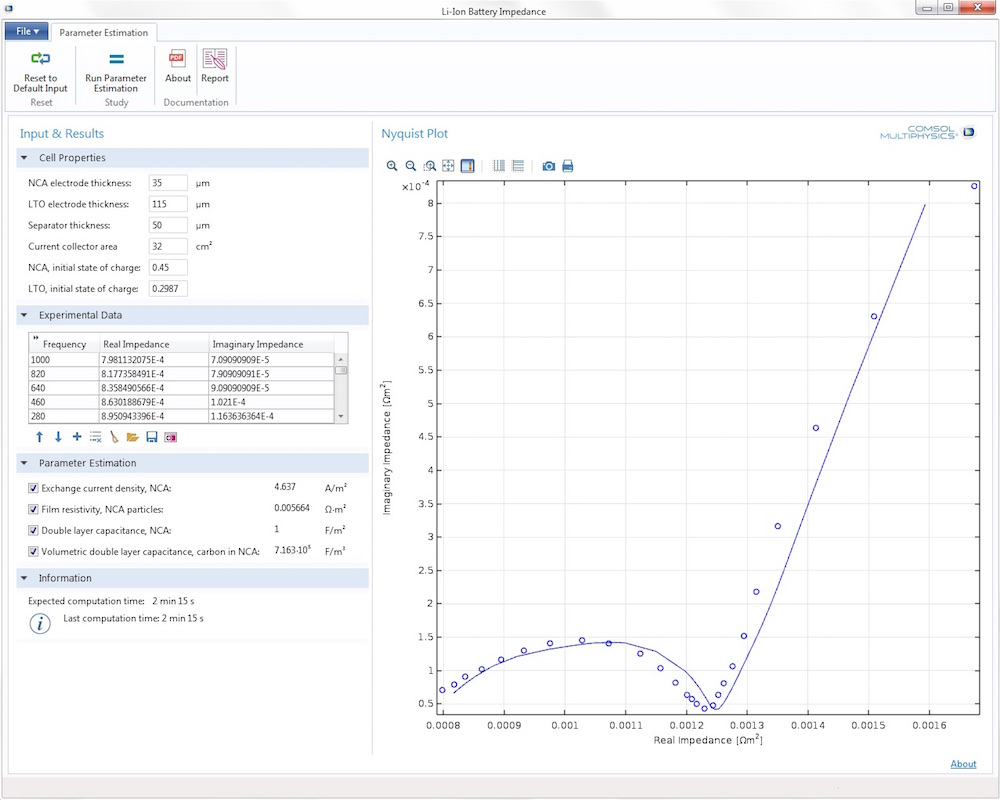## Studying Impedance to Analyze the Li-Ion Battery with an App

#####Tommy Zavalis July 14, 2015

Batteries generally operate through numerous processes that depend on even more parameters. How can you find out more about what’s going on within them? One approach is to look at the cell’s electrical impedance. The Lithium-Ion Battery Impedance demo app, available in the Application Gallery, can be used to interpret the impedance of a specific lithium-ion battery design with minimal effort. It can also help parameterize the system, a useful step for setting up accurate time-dependent models in the future.

### Impedance Spectroscopy: An Experimental Method

Electrochemical impedance spectroscopy (EIS) is a very widely used technique in electroanalysis. It is used to study the harmonic response of an electrochemical system. For batteries, a small, sinusoidal variation is applied to the potential difference between the two electrodes, and the resulting current is analyzed in the frequency domain in terms of impedance. Normally, the perturbation is applied about the open-circuit voltage.

In electrical analysis, the impedance is a complex quantity that includes a real and an imaginary component. The former corresponds to a resistance in-phase with the applied voltage; the latter is a reactance 90° out-of-phase with the applied voltage. The real and imaginary components of the impedance give information about the kinetic and mass transport properties of the cell, as well as its capacitive properties. By measuring impedance at a range of frequencies, the relative influence of the various constituent physics of the system can be interpreted as a function of their characteristic timescales.

### How to Model Impedance in a Lithium-Ion Battery

Several processes within a lithium-ion battery cell exhibit time-dependent responses that are detectable in the frequency domain. For a typical lithium-ion battery cell made up of two porous electrodes with a porous separator in between, as seen in the figure below, the following processes are accountable:

• Charge transfer reaction on the surface of the active electrode materials.
• Mass transport (diffusion and migration) in the electrolyte.
• Diffusion of lithium within the active electrode material particles.
• Change of double-layer charge on the active electrode material, electrical conductor, and other interfaces.
• Contact resistance between electrically conducting materials.Processes and materials in a lithium-ion battery cell.

The Lithium-Ion Battery interface easily accounts for all of these phenomena. In the electrolyte, both charge and mass balances are set up. In the active material particles, mass balances are solved. For instance, the charge transfer reaction can be modeled with Butler-Volmer kinetics. At the surface of all solid phase materials, double-layer currents and a film resistance can be introduced. All equations in the interface are based on the transient descriptions.

For impedance modeling, the COMSOL Multiphysics software automatically transforms these equations into the frequency domain and linearizes them around a given voltage and current. The linearization approach is in line with the harmonic interpretation of impedance data, and it can be used because the perturbation to the cell potential is chosen to be small.

### How Can Impedance Data Be Interpreted?

A common way to display the impedance of a system is with a Nyquist plot, in which the negative imaginary component of impedance is plotted against the real component of impedance. For a single porous electrode (see the figure above), the Nyquist plot often looks like that shown below.Nyquist plot and the contributions of various properties.

The semicircles within the mid-high frequency window display the charging of double layers on the materials within the electrode and the contribution of different resistances; these can be due to the electrode materials and the presence of resistive films, for instance. One of the semicircles gives an indication of the rate of the charge transfer reaction.

At lower frequencies, a “tail” is shown. The shape of the tail is principally affected by the diffusion within the electrolyte and active electrode materials. In essence, it is controlled by the diffusion coefficients and the particle size for the electrode material. The real impedance at the leftmost point in the Nyquist plot gives a measure of the ionic and electrical conductivity within the cell.

All in all, impedance supplies a considerable amount of information that a model can help to organize. One approach is to vary model parameters to pinpoint what affects the impedance and at what frequency, as seen in the figure below. Alternatively, one can fit the model to experimental impedance data through an optimization procedure and examine the optimized properties.Nyquist plots with the change of various parameters.

### The Lithium-Ion Battery Impedance App

With a simulation app, you can decipher experimental EIS measurements in a quick, straightforward manner. It functions by using experimental data from EIS measurements as input, simulates these measurements, and then fits the model to the experimental data through parameter estimation.

The studied battery cell design consists of the following components:

• Positive porous electrode: NCA (LiNi0.08Co0.15Al0.05O2) active material, electrical conductor, and binder.
• Negative porous electrode: LTO (Li4Ti5O12) active material, electrical conductor, and binder.
• Separator: Celgard 2325.
• Electrolyte: 1.2 M LiPF6 in EC:EMC (3:7 by weight).

The fitting is done to the measurements of the positive electrode at frequencies ranging from 10 mHz to 1 kHz.

The thicknesses of the electrodes and separator together with the current collector area and the initial state-of-charge of the electrodes can be varied in the Cell Properties section. An Experimental Data section enables you to import any measured impedance data that you want to investigate.

In the Parameter Estimation section, the selection of the estimated control parameters is made. Available parameters include the exchange current density, the resistivity of the resistive layer on the particles, the double-layer capacitance of NCA, and the double-layer capacitance of the carbon support in the positive electrode.

Here is what the user interface looks like when the battery design has been optimized:The lithium-ion battery impedance app.

In this case, the magnitudes of the optimized parameters tell us whether the associated processes are present. Is there any substantial charging of double layers? Is the resistance due to film resistance on the active particles significant? How fast is the charge transfer reaction? The parameters are also ready to be used for setting up, for instance, a time-dependent battery model of the system. You can also easily move on and benchmark different batteries or import impedance data from another battery cell, perhaps an aged one. With COMSOL Multiphysics, there are many possibilities!

### Join the Simulation Revolution: Run the Demo App Now

#### Older Post

Building an IR Microscope App at ZINK Imaging